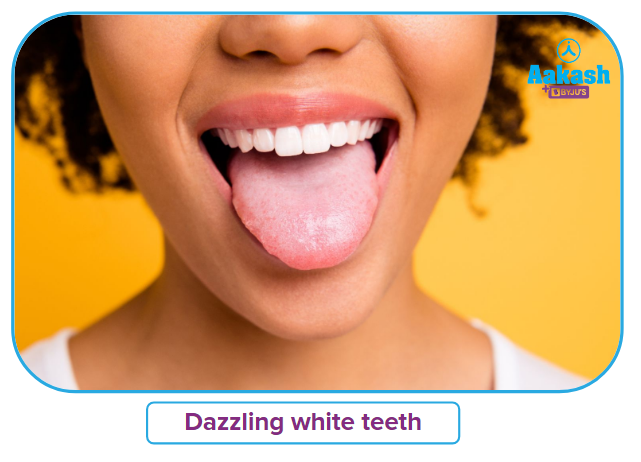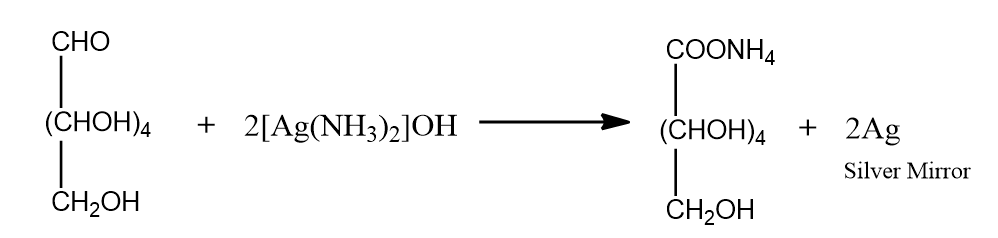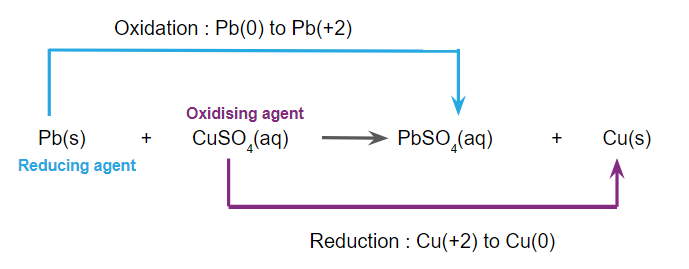• Call Now

1800-102-2727•

# Oxidation and Reduction-Oxidising and Reducing agents - definitions, examples, Practice problems

Our teeth can become stained over time from things like smoking, drinking coffee, red wine, and ageing.

Who wouldn't desire a bright, white smile?

One of the most common dental procedures each year is tooth whitening. There are numerous options available if you want to enhance the beauty of your smile. The terms "bleaching" and "whitening" are frequently used interchangeably.

Bleaching products contain peroxide (hydrogen peroxide or carbamide peroxide), an oxidising agent that eliminates stains from teeth's surface and interior. These products may make teeth appear lighter than they are naturally.

I hear you asking, "Wait a minute, what exactly are oxidising agents? Are there also reducing agents if there are oxidising agents? So what exactly are reducing agents?

Take a chill pill. Because the information on this content page has the answers to these queries.

So let's explore oxidising and reducing agents in further detail.• Oxidising Agents
• Examples of Oxidising Agents
• Reducing Agents
• Examples of Reducing Agents
• Understanding the Terms Involved
• Practice Problems
• Frequently Asked Questions - FAQ

### Oxidising Agents

• An oxidising agent (also known as an oxidizer or an oxidant) is a chemical species that tend to oxidise other substances, causing them to lose electrons and thus increase their oxidation state.
• Halogens, oxygen, and hydrogen peroxide are common oxidising agents.
• Oxidising agents typically exist in the highest possible oxidation states.
• Ions, atoms and molecules with a high affinity for electrons act as effective oxidising agents.
• The power of the oxidising agent increases with the increase in their electron affinity.
• Oxidising agents are electron acceptors. They have a great tendency to accept electrons and themselves undergo reduction.

### Examples of Oxidising Agents

1. There are numerous oxidising agents that are often utilised in both industrial settings and daily life. Examples include sulphuric acid (H2SO4), potassium nitrate (KNO3), and household bleach (NaClO).

Example: $Zn\left(s\right)+{H}_{2}{SO}_{4}\left(aq\right)\to Zn{SO}_{4}\left(aq\right)+{H}_{2}\left(g\right)$

In this equation, H2SO4 act as an oxidising agent. It oxidises Zn and itself gets reduced.

- - Oxidation

- Reduction

1. Oxygen is a highly reactive non-metal with effective oxidising characteristics that is a member of the periodic table's chalcogen group. Due to the high oxidising properties of oxygen in the air, metals typically react with oxygen to produce metal oxides.

Example: $2Cu\left(s\right)+{O}_{2}\left(g\right)\to 2CuO\left(s\right)$

In this equation, O2 acts as an oxidising agent. It oxidises Cu and itself gets reduced.

- Oxidation

- Reduction

1. Halogens have higher electronegativities than the other elements in their respective period and are hence said to have a greater ability to gain electrons. Halogens like iodine, bromine, chlorine, and fluorine are a few examples of effective oxidising agents. Fluorine is thought to have the highest electronegativity of all the elements, making it the most powerful oxidising agent.

Example:$2NaCl\left(aq\right)+{F}_{2}\left(g\right)\to 2NaF\left(aq\right)+{Cl}_{2}\left(g\right)$

In this equation, F2 act as an oxidising agent. It oxidises NaCl and itself gets reduced.

- Oxidation

- Reduction

1. Hydrogen Peroxide functions as a mild oxidising, cleaning, and bleaching agent.

Example: ${4H}_{2}{O}_{2}\left(aq\right)+PbS\left(s\right)\to {4H}_{2}O\left(aq\right)+PbS{O}_{4}\left(aq\right)$

In this equation, H2O2 act as an oxidising agent. It oxidises PbS and itself gets reduced.

- Oxidation

- Reduction

### Reducing Agents

• A reducing agent (also known as a reducer or a reducant) is a chemical species that tends to reduce other substances, causing them to gain electrons and thus decrease their oxidation state.
• Elements of groups 1 and 2 are common reducing agents.
• Reducing agents typically exist in the lowest possible oxidation states.
• Ions, atoms and molecules with a low affinity for electrons act as effective reducing agents.
• The power of the reducing agent decreases with the increase in their electron affinity.
• Reducing agents are electron donators. They have a great tendency to donate electrons and themselves undergo oxidation.

### Examples of Reducing Agents

1. Metals like Na, Fe, Zn, and Al, as well as non-metals like C, S, and H2 are examples of common reducing agents. Some substances, including hydracids, like HCl, HI, HBr, and H2S, act well as reducing agents.

Example: $2HCl\left(aq\right)+{F}_{2}\left(g\right)\to 2HF\left(aq\right)+{Cl}_{2}\left(g\right)$

In this equation, HCl act as a reducing agent. It reduces F2 and itself gets oxidised

- Oxidation

- Reduction

1. When added to solutions, lithium is believed to be a powerful reducing agent.

Example: $2Li\left(s\right)+{F}_{2}\left(g\right)\to 2LiF\left(s\right)$

In this equation, Li acts as a reducing agent. It reduces F2 and itself gets oxidised.

- Oxidation

- Reduction

1. Iodide salts are thought to be mild reducing agents. Iodine is produced when they interact with oxygen. These also have a variety of antioxidant qualities.

Example: ${Cr}_{2}{O}_{7}^{2-}+6{I}^{-}+{14H}^{+}\to 2{Cr}^{3+}+{3I}_{2}+7{H}_{2}O$

In this equation, ${I}^{-}$ acts as a reducing agent. It reduces ${Cr}_{2}{O}_{7}^{2-}$ and itself gets oxidised.

- Oxidation

- Reduction

1. Due to the presence of a free ketone group or a free aldehyde group, reducing sugars function similarly to reducing agents. The term "reducing sugars" refers to all monosaccharides as well as disaccharides, polysaccharides, and oligosaccharides.

Example:### Understanding the Terms Involved

Observe the given reaction. When lead (Pb) reacts with copper sulphate solution, lead (Pb) is converted to lead sulphate (PbSO4) and copper sulphate (CuSO4) to copper (Cu). There is an increase in the oxidation state of lead, confirming its oxidation, and a decrease in the oxidation state of copper, confirming its reduction.In a redox reaction, the oxidising agent undergoes reduction i.e. it gains electrons, and the reducing agent undergoes oxidation i.e. it loses electrons.

In the given reaction, the oxidising agent is copper sulphate (CuSO4) as copper (Cu) goes from +2 to 0 oxidation state i.e it accepts 2 electrons from lead (Pb) and undergoes reduction.

Similarly, the reducing agent in this reaction is lead (Pb) as it goes from 0 to +2 oxidation state i.e it donates 2 electrons to copper (Cu) and undergoes oxidation.

### Practice Problems

Q1. Which will behave as an oxidising agent in the given reaction?

$\mathbit{P}\mathbit{b}{\mathbit{S}\mathbit{O}}_{4}\left(\mathbit{a}\mathbit{q}\right)+\mathbit{Z}\mathbit{n}\left(\mathbit{s}\right)\to \mathbit{Z}\mathbit{n}{\mathbit{S}\mathbit{O}}_{4}\left(\mathbit{a}\mathbit{q}\right)+\mathbit{P}\mathbit{b}\left(\mathbit{s}\right)$

A. PbSO4(aq)
B. Zn(s)
C. Pb(s)
D. Both (A) and (C)

Solution: The oxidation state of Pb in PbSO4(aq) is +2, and the oxidation state of Pb in Pb(s) is 0 i.e. Pb2+ accepts two electrons from Zn and becomes Pb. Therefore Pb2+ (PbSO4) undergoes reduction, or in other words, it acts as an oxidising agent.

(reduced/oxidising agent)

The oxidation state of Zn in Zn(s) is 0 and the oxidation state of Zn in ZnSO4(aq) is +2 i.e. Zn donates two electrons to Pb2+ and becomes Zn2+. Therefore, Zn undergoes oxidation, or in other words, it acts as a reducing agent.

(oxidised/redducing agent)

Hence, PbSO4(aq) is the oxidising agent in the given reaction.

So, option A) is the correct answer.

Q2. Which of the following will act as a reducing agent in the given reaction?

$4\mathbit{H}\mathbit{N}{\mathbit{O}}_{3}\left(\mathbit{a}\mathbit{q}\right)+\mathbit{C}\mathbit{u}\left(\mathbit{s}\right)\to \mathbit{C}\mathbit{u}\left(\mathbit{N}{\mathbit{O}}_{3}{\right)}_{2}\left(\mathbit{a}\mathbit{q}\right)+{2\mathbit{N}\mathbit{O}}_{2}\left(\mathbit{g}\right)+{2\mathbit{H}}_{2}\mathbit{O}\left(\mathbit{a}\mathbit{q}\right)$

A. HNO3(aq)
B. Cu(s)
C. Cu(NO3)2
D. 2H2O(aq)

Solution: The oxidation state of N in HNO3(aq) is +5, and the oxidation state of N in NO2(g) is +4 i.e. N5+ accepts an electron from Cu and becomes N4+. Therefore N5+ (HNO3) undergoes reduction, or in other words, it acts as an oxidising agent.

(reduced/oxidising agent)

The oxidation state of Cu in Cu(s) is 0 and the oxidation state of Cu in Cu(NO3)2(aq) is +2 i.e. Cu donates two electrons and becomes Cu2+. Therefore, Cu undergoes oxidation, or in other words, it acts as a reducing agent.

(oxidised/reducing agent)

Hence, Cu (s) is the reducing agent in the given reaction.

So, option B) is the correct answer.

Q3. For the reaction $\mathbit{C}\mathbit{u}{\mathbit{C}\mathbit{l}}_{2}+{\mathbit{H}}_{2}\to 2\mathbit{H}\mathbit{C}\mathbit{l}+\mathbit{C}\mathbit{u}$, pick out the correct statement.

A. CuCl2 is reduced and H2 is oxidised.
B. CuCl2 is oxidised and H2 is reduced.
C. CuCl2 is both oxidised and reduced.
D. H2 is both oxidised and reduced.

Solution: The oxidation state of Cu in CuCl2 is +2, and the oxidation state of Cu in Cu is 0 i.e. Cu2+ accepts two electrons and becomes Cu. Therefore, Cu2+ (CuCl2) undergoes reduction, or in other words, it acts as an oxidisng agent.

(reduced/oxidising agent)

The oxidation state of H in H2 is 0, and the oxidation state of H in HCl is +1 i.e. H donates an electron and becomes H+. Therefore H (H2) undergoes oxidation, or in other words, it acts as a reducing agent.

(oxidised/reducing agent)

Thus, CuCl2 is reduced and H2 is oxidised in the given reaction.

So, option A) is the correct answer.

Q4. Which of the following will act as both a reducing agent and an oxidising agent in the given reaction?

$4{\mathbit{C}\mathbit{l}}_{2}\left(\mathbit{g}\right)+8{\mathbit{O}\mathbit{H}}^{-}\left(\mathbit{a}\mathbit{q}\right)\to {7\mathbit{C}\mathbit{l}}^{-}\left(\mathbit{a}\mathbit{q}\right)+\mathbit{C}\mathbit{l}{\mathbit{O}}_{4}^{-}\left(\mathbit{a}\mathbit{q}\right)+4{\mathbit{H}}_{2}\mathbit{O}\left(\mathbit{a}\mathbit{q}\right)$

A. Cl2(g)
B. Cl-(aq)
C. $Cl{O}_{4}^{-}\left(aq\right)$
D. All of these

Solution: The oxidation state of Cl in Cl2(g) is 0, and the oxidation state of Cl in Cl-(aq) is -1 i.e Cl accepts an electron and becomes Cl-(aq). Therefore, Cl undergoes reduction, or in other words, it acts as an oxidising agent.

(reduced/oxidising agent)

The oxidation state of Cl in Cl2(g) is 0, and the oxidation state of Cl in $\mathbit{C}\mathbit{l}{{\mathbit{O}}_{4}}^{-}\left(\mathbit{a}\mathbit{q}\right)$ is +7 i.e. Cl donates 7 electrons and becomes Cl+7(aq). Therefore, Cl undergoes oxidation, or in other words, it acts as a reducing agent.

(oxidised/reducing agent)

Hence, Cl2(g) gets reduced and oxidised, and can therefore act as both a reducing and an oxidising agent.

So, option A) is the correct answer.

## Frequently Asked Questions - FAQ

Q1. Is there a difference between a reducing agent and an anti-oxidant?
Antioxidant agents can stop a chain reaction by getting rid of the free radical intermediates. Antioxidants can be thought of as reducing agents since they are oxidised while acting as antioxidants. Examples include polyphenols, thiols and ascorbic acid.

Q2. What biological effects do oxidising agents have?
The harmful effects of oxidants include damage to lipids, proteins and DNA, as well as the acceleration of atherosclerosis, rheumatoid arthritis, and cataract development. Additionally, oxidants are to blame for the ischemia-reperfusion syndrome.

Q3. Why are metals referred to as reducing agents?
Because all metals are relatively electropositive and have low ionisation energies, they lose electrons relatively quickly. As a result of their tendency to donate electrons and become oxidised, metals act as reducing agents.

Q4.  What effect do oxidants have on respiration?
High doses of oxidants like ozone and nitrogen dioxide result in death from lung oedema. Worsening of asthma (acute effects) and effects on lung growth are well-established effects on the respiratory system (chronic effects). Long-term exposure to oxidising agents such as ozone has recently been linked to negative cardiovascular outcomes, including increased mortality.Talk to our expert
Resend OTP Timer =
By submitting up, I agree to receive all the Whatsapp communication on my registered number and Aakash terms and conditions and privacy policy